# First-order linear differential equation

## Definition

### Format of the differential equation

A first-order linear differential equation is a differential equation of the form:$\frac{dy}{dx} + p(x)y = q(x)$

where$p,q$ are known functions.

### Solution method and formula: indefinite integral version

Let$H(x)$ be an antiderivative for$p(x)$, so that$H'(x) = p(x)$. Then, we multiply both sides by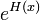$e^{H(x)}$. Simplifying, we get:$\frac{d}{dx}[e^{H(x)}y] = q(x)e^{H(x)}$

Integrating, we get: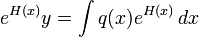$e^{H(x)}y = \int q(x)e^{H(x)} \, dx$

Rearranging, we get:$y = e^{-H(x)}\int q(x)e^{H(x)} \, dx$ where$H$ is an antiderivative of$p$.

In particular, we obtain that: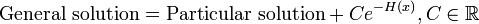$\mbox{General solution} = \mbox{Particular solution} + Ce^{-H(x)}, C \in \R$

The function$e^{H(x)}$ is termed the integrating factor for the differential equation because multiplying by this turns the differential equation into an exact differential equation, i.e., a differential equation to which we can apply integration on both sides.

### Solution method and formula: definite integral version

Suppose we are given the initial value condition that at$x = x_0, y = y_0$.

Let$H(x)$ be an antiderivative for$p(x)$, so that$H'(x) = p(x)$. Then, we multiply both sides by$e^{H(x)}$. Simplifying, we get:$\frac{d}{dx}[e^{H(x)}y] = q(x)e^{H(x)}$

Integrating from$x_0$ to (arbitrary)$x$, we get: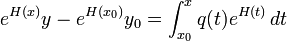$e^{H(x)}y - e^{H(x_0)}y_0= \int_{x_0}^x q(t)e^{H(t)} \, dt$

Thus, the general expression is:$y = e^{-H(x)}\left(e^{H(x_0)}y_0 + \int_{x_0}^x q(t)e^{H(t)} \, dt\right)$

## Examples

### Simple example

Consider the differential equation:$y' + y = e^{e^x}$

Here,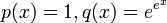$p(x) = 1, q(x) = e^{e^x}$. Take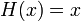$H(x) =x$ and get:$y = e^{-x} \int e^{e^x}e^x \, dx$

This gives: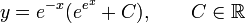$y = e^{-x}(e^{e^x} + C), \qquad C \in \R$

### Example that is better solved by subtitution

Consider: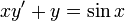$xy' + y = \sin x$

Divide both sides by$x$ to get:$y' + \frac{y}{x} = \frac{\sin x}{x}$

This is linear, with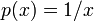$p(x) = 1/x$,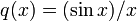$q(x) = (\sin x)/x$. Take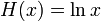$H(x) = \ln x$ and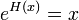$e^{H(x)} = x$ (see note):$y = \frac{1}{x} \int \frac{\sin x}{x} x \, dx$

This gives:$y = \frac{C - \cos x}{x}, \qquad C \in \R$

The linear method is unnecessary -- we divided and multiplied by$x$. A better solution would be to substitute$u = xy$ and get a separable differential equation.

### Example where a particular solution is obtained by inspection

Consider:$y' + y = \tan x + \tan^2x$

The linear method gives: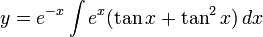$y = e^{-x} \int e^x(\tan x + \tan^2x) \, dx$

The integration is not easy. So, instead of trying to do the integration directly, we note that the answer is:$y = \mbox{Particular solution} + Ce^{-x}$

It thus suffices to find a particular solution. Inspection and guesswork gives a solution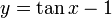$y = \tan x - 1$. The general solution is thus: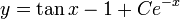$y = \tan x - 1 + Ce^{-x}$